HomeBlogGeneral25 Advanced Excel Formulas – A Must Know For All Professionals

# 25 Advanced Excel Formulas – A Must Know For All Professionals

Last updated:
1st Apr, 2023
Views
19 MinsView AllIf you find yourself often flexing your hands and wasting hours to do things manually in Excel, you are missing out on how powerful Excel can be if you know how to use it the right way. Microsoft Excel is known for its formulas that can work efficiently over large numbers of data in the cell, without much manual involvement needed in the process.

We have compiled 25 advanced excel formulas that have an uncanny ability to transform lengthy mundane, manual tasks into a few seconds of work. These are formulas that every professional should have on their fingertips to save their own precious time, or to impress their boss at a critical moment.

Individuals who wonder about how many formulas in excel are there can go through the list and do tell us your favorite!

##### 1. INDEX MATCH

Formula = INDEX(C3:E9,MATCH(B13, C3:C9,0),MATCH(B14,C3:E3,0))

An excellent alternative to the VLOOKUP or HLOOKUP formula on Excel that has some drawbacks for performing lookup tasks. The INDEX MATCH is a combination formula of 2 separate functions:

INDEX: This formula returns the value of a cell in a table based on the column and the row number.

MATCH: This formula returns the position of a cell in a row or column.

An example of the INDEX and MATCH formulas combine is: When you are looking up and return a person’s height based on their name, you can use this formula to change both the variables (in this case, name and height) using the INDEX MATCH formula.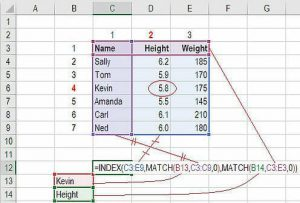Source

Let us break it down step-by-step:

How to Combine INDEX and MATCH

• Type INDEX
• Select Area you want to INDEX
• Lock the area with F4
• Find the Row you want to look for the data from
• Type MATCH with the series of information and lock the area with F4
• Type 0 for the exact match, close the brackets
• Hit Enter

Now you have a fully dynamic and functional set of columns and rows in the sheet where all combing through the cells and rows for the right data is done automatically.

##### 2. AVERAGE

To perform the average formula to find the mean of any range of numbers, here are the steps you need to follow:

Enter the values, cells or scale of cells that you’re calculating in the format.

The formula shall begin with =AVERAGE(number1, number 2, etc.)

If you want to perform the average of a range of cells in the sheet, here are the steps you need to follow:

• Enter the values, cells or scale of cells that you’re calculating in the format, just like you did above
• The formula shall be like =AVERAGE(Start Value: End Value).

If you are wondering how to enter the values for the region of cells, you can select the area on your sheet with your mouse and lock it in with F4. This shall make Excel do the rest of the work for you without doing any sum and division of the figure with the number of items in the group.

##### 3. SUMIF

This formula in excel is denoted as SUMIF(range, criteria, [sum range]). This would result in the sum of the values within the desired Range of cells that would meet the requirements set by you. For example, =SUMIF(C3: C12, “>70,000) would return the sum of values between the cells of C3 and C12 from only the cells that have the value more than 70,000.

In the case of finances, salary, or even cost calculations, you need the value of leads who are associated with specific employees determined by the condition set by you. Doing this manually is very time consuming and slows down things.

The formula for the above condition can be =SUMIF(range, criteria, [sum_range]), where Range can be defined as the Range of the sheet that you need to choose the values from.

[sum_range] is defined as the additional or optional Range that you’re going to add up in addition to the first Range entered.

Here’s an example: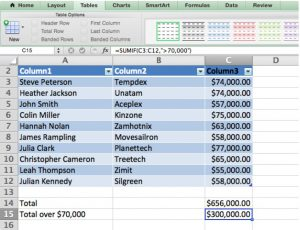Source

##### 4. OFFSET COMBINED WITH SUM

Alone, the OFFSET function may not be handy, but when combined with other services, you can get a complex formula with faster results if you want to create a dynamic role which can sum any variable number of cells, the regular SUM formula, which is static needs to be combined with the OFFSET function.

So for finding out the sum of values in variable cells, the formula should be:

=SUM(B4:OFFSET(B4,0,E2-1))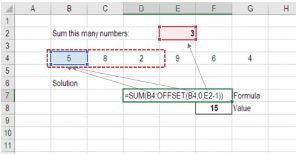Source

Here the ending reference of the SUM Function gets replaced with the OFFSET function. The SUM formula starting in B4 ends with a variable and is an OFFSET formula starting at B4, continuing by the value in E2 (“3”) minus one. This helps the method to move over two cells and summing three years of data. In the above reference, you can see that the cell F7 holds the sum of the cells B4: D4 = 15.

##### 5. IF AND

This formula comes handy in situations when you need to create certain conditions to comb through a heap of data. The IF statement helps in using the advanced excel IF function for creating a new field based on these conditions on an already existing track.

Anybody who has devoted much time doing different types of financial models understands how complex nested IF formulas can be. Combining IF with the AND or the OR function can help keep the formulas easier to review and understand. So, this function is one of the easy-to-use advanced excel formulas.

For example, if you want to mark the employees with salaries above 50K and employee ID greater than 3, then the formula will be:

IF(AND(E4>3,F4>50000)1,0)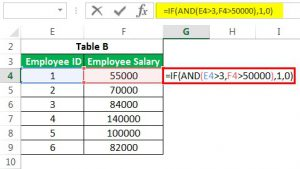Source

Since there are no real cases in the above data, the formula would return the value 0.

##### 6. CONCATENATE

Concatenate is not a function on its own, but it’s only a creative way of combining information from various cells, thus making the worksheets more dynamic.  It is an efficient tool for financial analysts performing financial modeling. So, financial analysts consider Concatenate function as one of the most useful advanced excel formulas.

If you have a lot of text strings in your datasheet and would like to make the data appear in one line, this formula is your go-to. For example, if you want to represent the employee ID and Employee name in a single column, the method should be:

=CONCATENATE(B3, C3)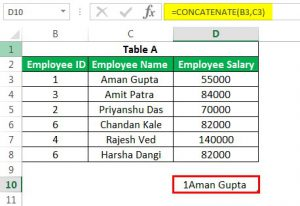Source

##### 7. PMT

The reason to name the function PMT is that it calculates the payment amount.

This function will help you figure out the future payments due for a loan, given a specific interest rate, principal amount, and duration of the loan. Here is what you need to begin:

• The term of the loan
• The starting principal (money) of the loan
• The future value
• The type of loan
• Compulsory Parameters in the PMT formula:RateNperPvOptional Parameters in the PMT formula:

[Fv]

[Type]

Now, if you want to find the monthly payment for a principal amount, the formula for the same will be:

= PMT (rate, per, PV, [fv], [type])

Let us understand this with an example: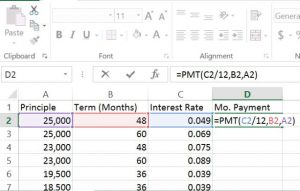Source

=PMT(C2/12.B2.A2)

And the best part is, you can drag the lower right corner of the PMT cells across the fields to automatically calculate the monthly payment amount for all the fields!

##### 8. TRIM

This is one of the most used formulas in excel that cleans any unwanted space in the fields. For example: If you need to remove the spaces at the beginning of some name, you can do that by using the TRIM function:

=TRIM(C6)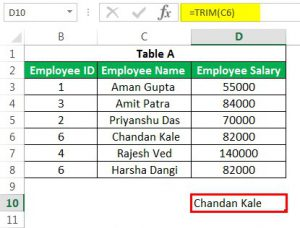Source

This would return you with the value of Chandan Kale without any extra spaces attached to the front or the end.

##### 9. LEN

This is a function which tells you the number of characters in a string of text. One of the most exciting ways to use this is, for example, if you want to highlight the donors who donated over \$1,000 by counting the number of digits in the donating column, the formula will be:

=LEN(B2)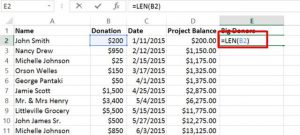Source

And if you want to highlight all the cells in the Donation column, then you need to:

• Select the Home tab in the menu
• Click on Conditional Formatting (in the toolbar)
• Select Use a Formula to determine which cells to format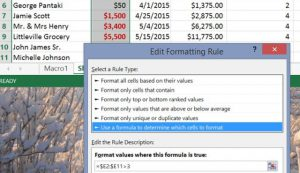Source

Here, if you make the condition “>3”, then anything over \$1,000 would receive the unique formatting, that you can choose by clicking the Format option.

##### 10. CHOOSE

This is a great function for scenario analysis in financial modelling. It allows you to pick between a specific number of options and return the “choice” which you’ve selected. For example, if you have three different values for revenue growth next year based on assumptions, using the CHOOSE function, you can return the amount according to your requirement.

The formula is:

=CHOOSE(C7,D3,D4,D5)

### How is the ‘CHOOSE’ Function useful in Excel?

When searching for a value equivalent to the index number or implementing the Vlookup function, you will realize that you have to pick the data from the left side because the Vlookup function works on the lookup value’s right side. Such problems can be solved through the CHOOSE function in excel. Therefore, it is one of the important functions in the advanced excel formulas list.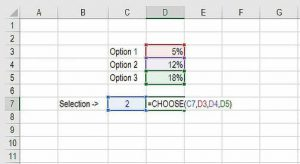Source

##### 11. CELL, LEFT, MID, and RIGHT

All the above functions can be combined in many ways to get some advanced formulas.

• The CELL function is used to return different types of information about the contents of the cell
• The LEFT service is used to replace text from the beginning of the cell.
• The MID function can return text from any of the starting points of the cell.
• The RIGHT function only returns text from the end of the cell.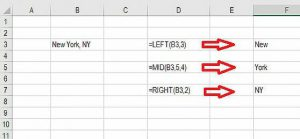Source

##### 12. XNPV and XIRR

For all the analysts who come across investment banking, equity research, or any other area of corporate finance that requires discounting cash flows, then these formulas are sure to save you a lot of time and grumbling!

XNPV and XIRR help you to include specific dates for each cash flow that is being discounted.  Excel’s basic NPV and IRR formulas assume that the periods between cash flow are the same.  Analysts may encounter situations where cash flows are not scheduled evenly. In that case, the XNPV and XIRR formulas can solve the problem; therefore, they are considered in the advanced excel formulas list.

For example, if you want to calculate the Present Net Value (NPV) for any investment using a specified rate of discounting and cash flows occurring irregular intervals, use the following function.

=XNPV(discount rate, cash flows, dates)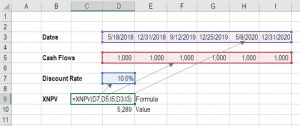Source

On the other hand, the XIRR function tells you the internal rate of return (where outflow = inflow) for a series of cash flows occurring at irregular intervals, such as: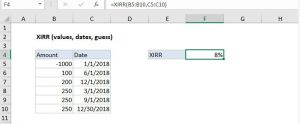##### 13. COUNTA ( )

This Microsoft Excel function counts the number of cells (whose values are not empty) and the number of value arguments given.  Note that a value argument is a parameter, not a cell or range of cells.

It is a built-in Excel function characterized as a Statistical Function. Moreover, it can be used as a worksheet function (WS). When working as a WS function, it can be applied as part of formula within a worksheet cell.

Note:

• If the argument is a cell and it is not empty, its value is considered ‘1’.
• If the argument is a value, not a cell or range, its value is considered ‘1’.
• If the argument is a range, the value of each cell in the range which is not empty is considered ‘1’.

Analysts are often found on their toes struggling to find the number of cells with values (numbers, errors, text, logical values) and those that are empty. The COUNTA( ) function can help you find out the name of non-empty cells in a selected range. For example, the formula for counting values for a data sheet having columns A1-A10 is:

=COUNTA(A1: A10)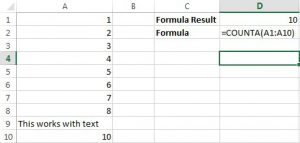Source

##### 14. FV

This formula comes in handy if you are looking to invest money in something and to know its worth. The requirements of the FV formula are:

• The interest rate of the loan
• Number of Payments
• The Payment for each period
• Current Starting Balance
• Type of Loan

For example, if you want to compare several blank CDs and you have a \$20,000 inheritance to invest in a CD. The interest rates are represented in the decimal format; payments are zero. The formula for the scenario will be:

=FV(A2/12,B2,C2,D2)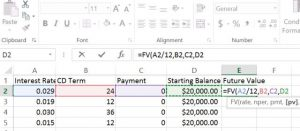Source

The results will be: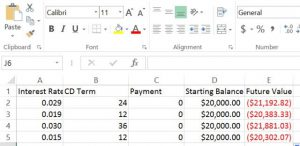Source

##### 15. RANDBETWEEN

RANDBETWEEN is a volatile function, which will alter the values created every time you newly define the formula on the same range. It generates a single random number at a single iteration between a specified range of numbers.

For example, for random numbers between 1 and 6, this function generates only integer random numbers by default.

This function helps to select a number within a predefined range of numbers randomly. Once you put the lowest and the highest numbers in the formula, Excel can choose the right data from the fields to which the names in the Range are attached and randomly pick from them. The method for the scenario is:

=RANDBETWEEN(starting point, ending point)

In this syntax,

Starting point: A required argument that defines the smallest value that the function can return

ending point: A required argument that defines the largest value that the function can return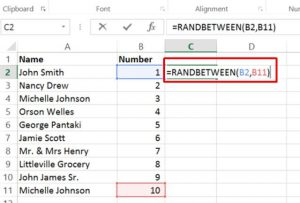Source

##### 16. SMALL

The SMALL function in Excel returns numeric values based on the position of the value in a list which is ranked by importance. This function helps to retrieve the “nth smallest values” from an array or Range of cells like the smallest value, 2nd lowest value, 3rd lowest value, etc.

The syntax for the formula is

=SMALL (times,range)

For example,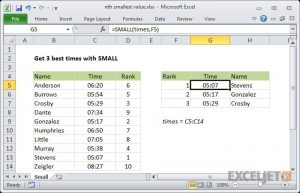Source

As the SMALL function is automatic, you need to supply a range and an integer for ‘nth’ to specify the ranked value. The official names for these arguments are ‘array’ and ‘k’.

Important Points to Remember about SMALL function:

• It ignores text values and only considers numerical values.
• It returns an error if no numerical values are available in the list.
• In the case of duplicates, the first value is considered the smaller one.
• K must be numeric; else, it returns the error as #VALUE!
• The given range must not be empty.
• When finding only the least value, you can use the MIN Function. It only finds the first smallest value.
• Although SMALL ignores text values, if any errors exist, it returns the result as #DIV/0!
• The SMALL function can be used with several other functions to find the Nth values
• When used with other functions, it works as an array formula
##### 17. QUARTILE

This function returns the quartile (each of four equal groups) in a given set of data and can return minimum value, first quartile, second quartile maximum value. This function brings the quartile amount of the fields in an array. The function returns a numerical value according to the requested percentile.

Syntax: =QUARTILE (array, quart)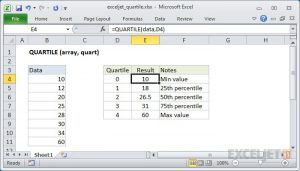Source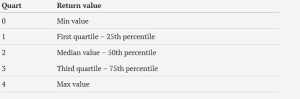Uses of QUARTILE function:

This built-in function in Excel falls under the Statistical functions category. It is alternatively known as a Worksheet function in excel. Working as a worksheet function can be used as a part of the formula within a cell of a worksheet.

##### 18. XLOOKUP

It is is one of the print functions. This function is available in the Excel 2021 and Excel for Microsoft 365. This is one of the advance excel formulas is the refined version of VLOOKUP. This formula can be used to scan the list, find the position value,  and return the value from the corresponding column in the same row.

##### 19. VLOOKUP

Another one of the advance excel formulas is VLOOKUP, which has been a significant function since forever. It can also be used to scan the list in a column and return the matching value from any column in the same row. This advance excel formula is can make a really big difference if you know VLOOKUP.

##### 20. FILTER

This is one of the most requested function which is used to filter a dataset in Excel and extract the useful information. This is one of the advanced excel formulas which is used to quickly filter the records to find any specific information.

##### 21. SORT and SORTBY

With the help of the excel formulas SORT and SORTBY the users can easily sort the data based on the desired column and row. It gives a good flexibility to choose any order based on preference. Moreover, the SORTBY formulas allows the users to sort the data based on multiple columns.

##### 22. UNIQUE

In the excel formulas list the function of UNIQUE has to be of mention. The UNIQUE function helps in giving the list of unique names. Before this function, there was a lot of labour involved in removing the duplicate functionality and using the advanced filter functionality.

##### 23. TEXTJOIN

The advanced excel of TEXTJOIN is one of the relatively new function which has come into picture recently in Excel 2019. As the name suggests, the formula can be used to quickly combine the content of a selected range of cells without the need of craetion for the concatenate formula.

##### 24. FIND/ SEARCH

This is one of the excel advanced formulas which are used to search for a given a text in a cell and helps to return to the starting position of a string which the users searched for.  With the use of LEFT/RIGHT/MID, one can do some serious manipulation and make the most out of the function.

##### 25. REPLACE/SUBSTITUTE

As the name suggests, these functions clearly help to find some text in a text free space and replace or substitute with the desired data. In the advanced excel formulas list, this function had to be there. REPLACE function can help to identify the starting position and the total characters one wants to replace with the specified text string.

## CONCLUSION:

Microsoft Excel is quite inevitable when it comes to the 21st-century workplace, but the trick is that it doesn’t have to be so daunting. Make it your friend, and watch your productivity shoot up!Blog Author
We are an online education platform providing industry-relevant programs for professionals, designed and delivered in collaboration with world-class faculty and businesses. Merging the latest technology, pedagogy and services, we deliver an immersive learning experience for the digital world – anytime, anywhere.
Get Free Consultation

Select CourseSelectBy clicking 'Submit' you Agree to

1Why is a spreadsheet so important?

Before acknowledging the use, let us first understand what a spreadsheet is. It is a computer program where data can be stored in the form of rows and columns. The data need not be perforce in numerical form. Any data recorded in a spreadsheet can be laid out, classified, and exhibited in the manner desired using the filter data option. In numerical data, the use of spreadsheets increases manifold. Aggregation, subtraction, multiplication, division, average, mean, mode, median, validatory conditions, etc., can all be done in a spreadsheet. To use it in an advanced way, knowledge of formulas is germane, which can help in evaluating and depicting the data in graphical format as well.

2What are the alternatives to excel?

MS Excel is an application launched in 1985, has a pervasive presence in the market, and is even now used by more than 1 out of 8 people on the planet. The application that instigated spreadsheets at a substantial scale can not be superseded nimbly but has undeniably gained many competitors. To name a few, Google sheets, Zoho Sheets, Apache OpenOffice Calc., LibreOffice Calc, and WPS Office spreadsheets. The increasing use of the Internet has made people use the services of spreadsheets digitally, without downloading the application on computers or cellphones. Few online spreadsheets are Confluence spreadsheets, Airtable, and EtherCalc.

3In which field spreadsheet is used?

Any field which entails maintaining a database uses a spreadsheet. The use of spreadsheets is inevitable in today’s world. Look for any industry, manufacturing, or service; they all have data that is required to be put down in an organised construction and need analysis at some juncture. Look for any profession, doctors who need to record the details of their patients, accountants who need to register their client details, company’s data regarding production, sales, cost, revenue, etc. Financial advisors analyse the data by marking the critical parameters and evaluating their performance, etc. This whole process, in whichever field, is made easy with the use of spreadsheets.

4Why is a spreadsheet so important?

Before acknowledging the use, let us first understand what a spreadsheet is. It is a computer program where data can be stored in the form of rows and columns. The data need not be perforce in numerical form. Any data recorded in a spreadsheet can be laid out, classified, and exhibited in the manner desired using the filter data option. In numerical data, the use of spreadsheets increases manifold. Aggregation, subtraction, multiplication, division, average, mean, mode, median, validatory conditions, etc., can all be done in a spreadsheet. To use it in an advanced way, knowledge of formulas is germane, which can help in evaluating and depicting the data in graphical format as well.

5What are the alternatives to excel?

MS Excel is an application launched in 1985, has a pervasive presence in the market, and is even now used by more than 1 out of 8 people on the planet. The application that instigated spreadsheets at a substantial scale can not be superseded nimbly but has undeniably gained many competitors. To name a few, Google sheets, Zoho Sheets, Apache OpenOffice Calc., LibreOffice Calc, and WPS Office spreadsheets. The increasing use of the Internet has made people use the services of spreadsheets digitally, without downloading the application on computers or cellphones. Few online spreadsheets are Confluence spreadsheets, Airtable, and EtherCalc.

6In which field spreadsheet is used?

Any field which entails maintaining a database uses a spreadsheet. The use of spreadsheets is inevitable in today’s world. Look for any industry, manufacturing, or service; they all have data that is required to be put down in an organised construction and need analysis at some juncture. Look for any profession, doctors who need to record the details of their patients, accountants who need to register their client details, company’s data regarding production, sales, cost, revenue, etc. Financial advisors analyse the data by marking the critical parameters and evaluating their performance, etc. This whole process, in whichever field, is made easy with the use of spreadsheets.

## Suggested Blogs

197598
Summary: In this article, you will learn about the top 7 courses after 12th commerce without Maths. B.Com (Bachelor of Commerce) B.Com (H) (Bachelor